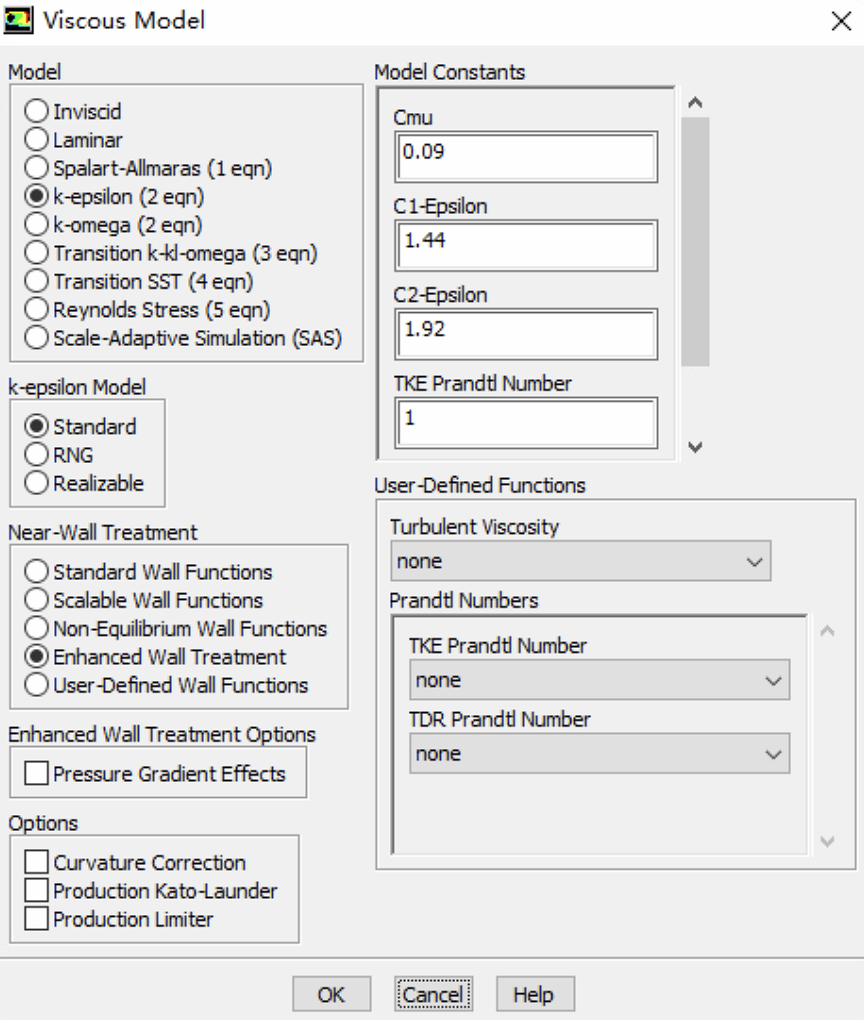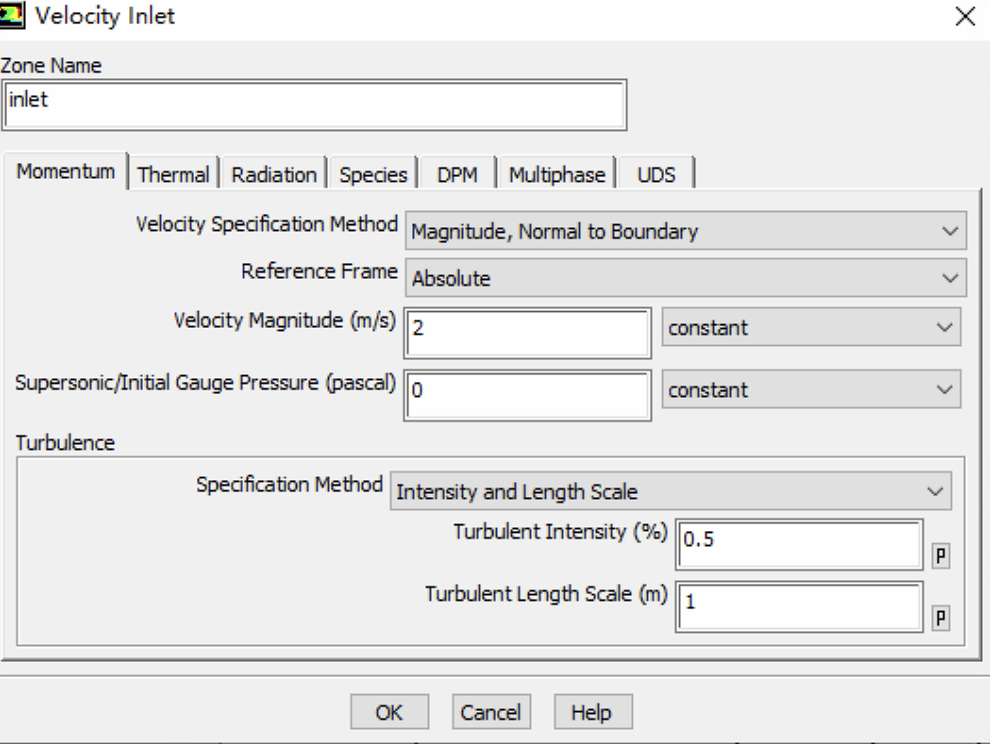# 槽道流使用fluent数值模拟入口边界条件设置

• 槽道流使用fluent数值模拟，2d模型，基于标准k-ε湍流模型，考虑使用增强壁面函数，选择速度边界条件，想咨询一下各位大神，速度入口边界条件这里对应的湍流强度和长度尺度应该怎么设置？使用增强壁面函数对应处理近壁面，这里选择是否合理呢，对于槽道流，壁面函数这样选择是否合理？• 来源：fluent help
Turbulence Intensity
$$I \equiv \frac{u^{\prime}}{u_{a v g}}=0.16\left(R e_{D_{H}}\right)^{-1 / 8}$$

Turbulence Length Scale
$$\ell=\frac{0.07 L}{C_{\mu}^{3 / 4}}$$

Turbulent Kinetic Energy
$$k=\frac{3}{2}\left(u_{a v g} I\right)^{2}$$

Turbulent Dissipation Rate
$$\varepsilon=\frac{k^{3 / 2}}{l}$$

• @veen 谢谢，我学习一下